Trigonometric Ratios - Introduction to Trigonometry, CBSE, Class 10, Mathematics

# Trigonometric Ratios - Introduction to Trigonometry, CBSE, Class 10, Mathematics - Notes - Class 10

 1 Crore+ students have signed up on EduRev. Have you?

INTRODUCTION
Trigonometry is the branch of Mathematics which deals with the measurement of angles and sides of a triangle.

The word Trigonometry is derived from three Greek roots : 'trio' meaning 'thrice or Three', 'gonia' meaning an angle and 'metron' meaning measure. Infact, Trigonometry is the study of relationship between the sides and the angles of a triangle.

Trigonometry has its application in astronomy, geography, surveying, engineering and navigation etc. In the past, astronomers used it to find out the distance of stars and plants from the earth. Even now, the advanced technology used in Engineering are based on trigonometrical concepts.

In this chapter, we will define trigonometric ratios of angles in terms of ratios of sides of a right triangle. We will also define trigonometric ratios of angles of 0°, 30°, 45°, 60° and 90°. We shall also establish some identities involving these ratios.

HISTORICAL FACTS

their innovation in the use of size instead the use of chord. The most outstanding astronomer has been Aryabhatta. Aryabhatta was born in 476 A.D. in Kerala. He studied in the university of Nalanda. In mathematics, Aryabhatta's contribution are very valuable. He was the first mathematician to prepare tables of sines. His book 'Aryabhatiya' deals with Geometry, Mensuration, Progressions, Square root, Cube root and Celestial sphere (spherical Trigonometry). This work, has won him recognition all over the world because of its logical and unambiguous presentation of astronomical observations.

Aryabhatta was the pioneer to find the correct value of the constant π with respect to a circle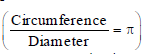upto four decimals as 3.1416. He found the approximate value of π and indirectly suggested that π is an irrational number. His observations and conclusions are very useful and relevant today.
Greek Mathematician Ptolemy, Father of Trigonometry proved the equation sin2A + cos2A = 1 using geometry involving a relationship between the chords of a circle. But ancient Indian used simple algebra to calculate sin A and cos A and proved this relation. Brahmagupta was the first to use algebra in trigonometry. Bhaskarcharaya II (1114 A.D.) was very brilliant and most popular Mathematician. His work known as Siddhantasiron mani is divided into four parts, one of which is Goladhyaya's spherical trigonometry.

BASE, PERPENDICULAR AND HYPOTENUSE OF A RIGHT TRIANGLE

In ΔABC, if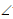B = 90°, then :

ForA, we have;

Base = AB, Perpendicular = BC and Hypotenuse = AC.

ForC, we have;

Base = BC, Perpendicular = AB and Hypotenuse = AC.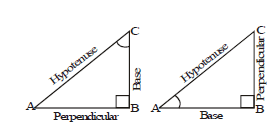So, in a right angled triangle, for a given angle,
(i) The side opposite to the right angle is called hypotenuse.
(ii) The side opposite to the given angle is called perpendicular.
(iii) The third side (i.e., the side forming the given angle with the hypotenuse), is called base.

TRIGONOMETRICAL RATIOS (T-RATIOS) OF AN ANGLE

In ΔABC, letB = 90° and letA be acute.
ForA, we have;
Base = AB, Perpendicular = BC and Hypotenuse = AC.
The T-ratios forA are defined as :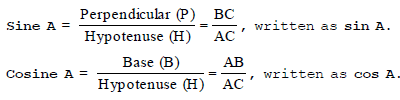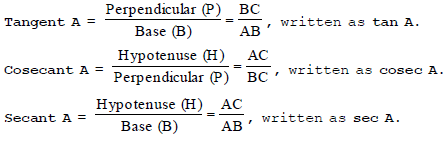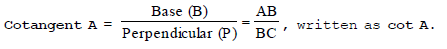Thus, there are six trigonometrical ratios based on the three sides of a right angled triangle.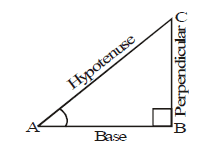Aid to Memory : The sine, cosine, and tangent ratios in a right triangle can be remembered by representing
them as strings of letters, as in SOH-CAH-TOA.

Sine = Opposite / Hypotenuse
The memorization of this mnemonic can be aided by expanding it into a phrase, such as "Some Officers Have
Curly Auburn Hair Till Old Age".

RECIPROCAL RELATIONS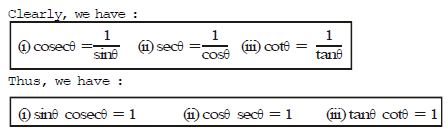QUOTIENT RELATIONS
Consider a right angled triangle in which for an acute angle θ, we have :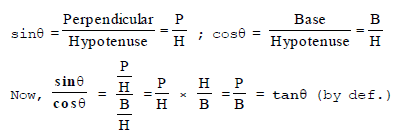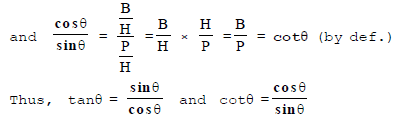POWER OF T-RATIOS

We denote :
(i) (sinθ)2 by sin2θ; (ii) (cosθ)2 by cos2θ; (iii) (sinθ)3 by sin3θ; (iv) (cosθ)3 by cos3θ and so on

REMARK : (i) The symbol sin A is used as an abbreviation for 'the sine of the angle A'. Sin A is not the product of 'sin' and A. 'sin' separated from A has no meaning. Similarly, cos A is not the product of 'cos' and A. Similar interpretations follow for other trigonometric ratios also.
(ii) We may write sin2 A, cos2 A, etc., in place of (sin A)2, (cos A)2, etc., respectively. But  cosecA = (sin A)–1 ≠ sin–1A (it is called sine inverse A). sin–1A has a different meaning, which will be discussed in higher classes. Similar conventions hold for the other trigonometric ratios as well.
(iii) Since the hypotenuse is the longest side in a right triangle, the value of sin A or cos A is always less than 1 (or, in particular, equal to 1).

Ex.1 Using the information given in fig. write the values of all trigonometric ratios of angle C

Sol. Using the definition of t-ratios,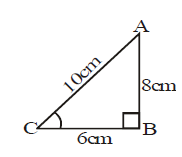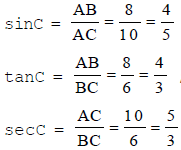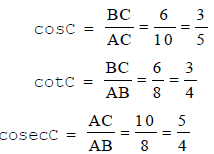Ex.2 In a right ΔABC, ifA is acute and tan A = 3/4 , find the remaining trigonometric ratios ofA.
Sol. Consider a ΔABC in whichB = 90°.

ForA, we have :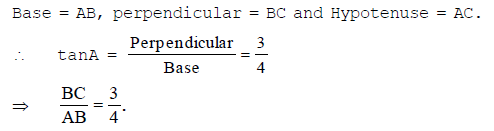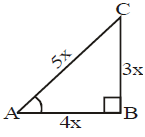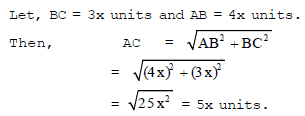Ex.3 In a DABC, right angled at B, if tan A  = 1/√3, find the value of

(i) sinA cosC + cosA sinC

(ii) cosA cosC – sinA sinC.

Solution: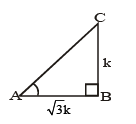tan = BC/AB = 1/√3

∴ AB : BC = 1:√3

Let BC = k and AB = √3k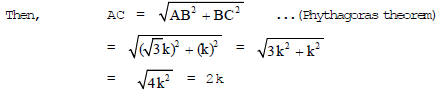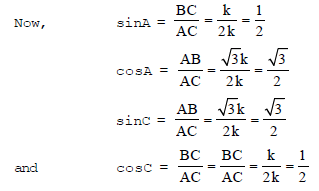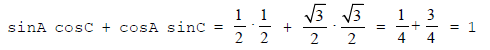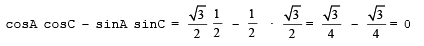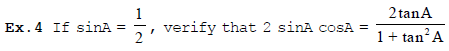Sol. We know that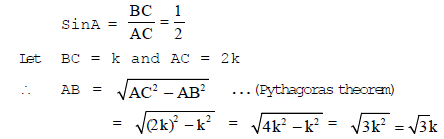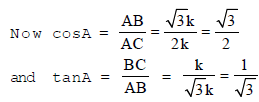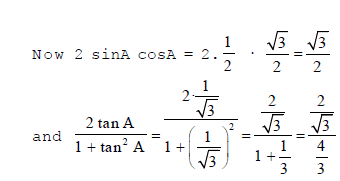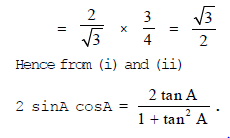Ex.5 In ΔPQR, right angled at Q, PR + QR = 25 cm and PQ = 5 cm. Find the value of sinP, cosP and tanP.
Sol. We are given
PR + QR = 25 cm
∴ PR = (25 – QR) cm
By Pythagoras theorem,
PR2 = QR2 + PQ2
or (25 – QR)2 = QR2 + 52
or 625 + QR2 – 50 QR = QR2 + 25
or 50 QR = 625 – 25 = 600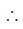QR = 12 cm.
and PR = (25 – 12) cm = 13 cm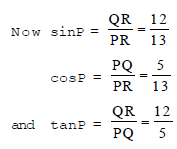The document Trigonometric Ratios - Introduction to Trigonometry, CBSE, Class 10, Mathematics - Notes - Class 10 is a part of Class 10 category.
All you need of Class 10 at this link: Class 10
 Use Code STAYHOME200 and get INR 200 additional OFF

Track your progress, build streaks, highlight & save important lessons and more!

,

,

,

,

,

,

,

,

,

,

,

,

,

,

,

,

,

,

,

,

,

,

,

,

,

,

,

,

,

,

;